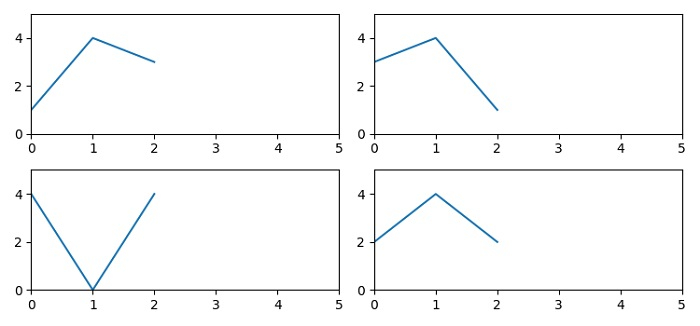# Setting the same axis limits for all subplots in Matplotlib

To set the same axis limits for all subplots in matplotlib we can use subplot() method to create 4 subplots where nrows=2, ncols=2 having share of x and y axes.

## Steps

• Set the figure size and adjust the padding between and around the subplots.

• Add a subplot to the current figure at index 1.

• Set the x and y axes view limit using set_xlim() and set_ylim() methods.

• Plot a line on axis 1 (step 2).

• Add a subplot to the current figure at index 2 with the same limit (step 3).

• Plot a line on axis 2.

• Add a subplot to the current figure at index 3 with the same limit (step 3).

• Plot a line on axis 3.

• Add a subplot to the current figure at index 4 with the same limit (step 3).

• Plot a line on axis 4.

• To show the figure, use show() method.

## Example

from matplotlib import pyplot as plt
plt.rcParams["figure.figsize"] = [7.50, 3.50]
plt.rcParams["figure.autolayout"] = True
ax1 = plt.subplot(2, 2, 1)
ax1.set_xlim(left=0, right=5)
ax1.set_ylim(bottom=0, top=5)
ax1.plot([1, 4, 3])
ax2 = plt.subplot(2, 2, 2, sharey=ax1, sharex=ax1)
ax2.plot([3, 4, 1])
ax3 = plt.subplot(2, 2, 4, sharey=ax1, sharex=ax1)
ax3.plot([2, 4, 2])
ax4 = plt.subplot(2, 2, 3, sharey=ax1, sharex=ax1)
ax4.plot([4, 0, 4])
plt.show()

## Output